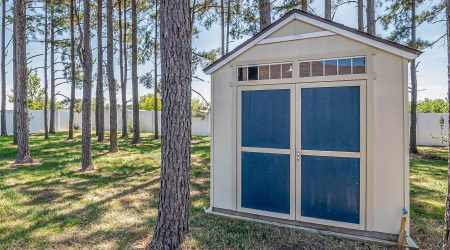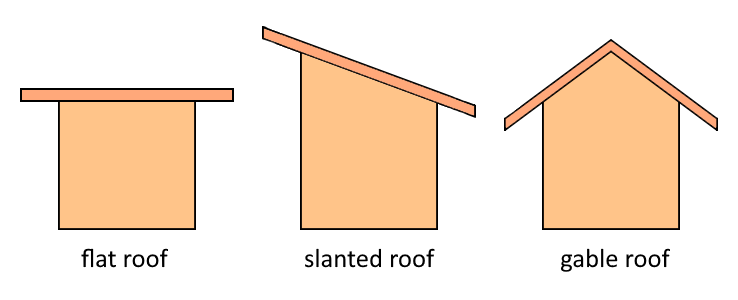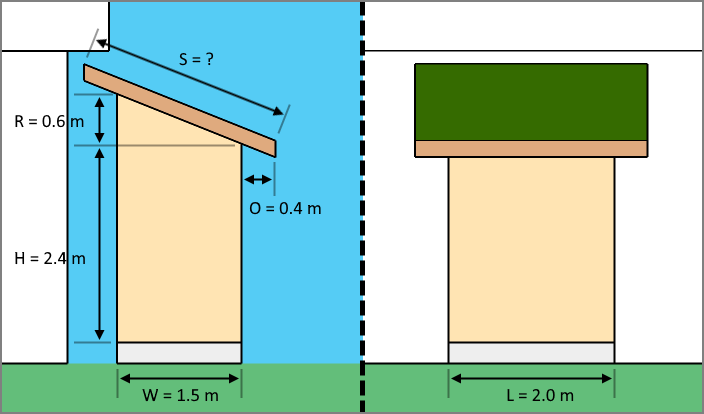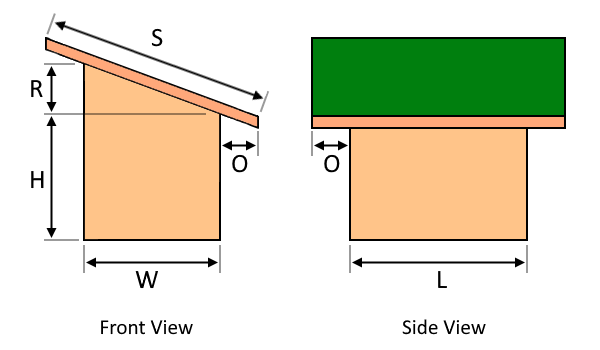# DIY Shed Cost Calculator

Created by Kenneth Alambra
Reviewed by Wojciech Sas, PhD and Jack Bowater
Last updated: Jun 05, 2023

This DIY shed cost calculator will help you estimate the cost to build a DIY shed depending on the dimensions you would like it to be and the type of roofing you decide your shed should have. This tool also works as a shed roof rafter span calculator and a general shed material calculator, whether you plan to build your own storage shed or want to learn something about sheds in general.

In this tool, you will learn about the basics of building a shed and the different factors that affect the number of materials you need when building a shed. You will learn how to perform shed material calculations for a flat-roof shed, a slant-roof shed, or a gable-roof shed. Keep on reading to start learning!

## The importance of building a shed (backyard shed, garden shed, or storage shed)

Building a shed gives us a little bit of additional storage space for our things at home. If you like spending your time taking care of your lawn or your outdoor plants, a garden shed would be a great fit for you, especially if you start to invest in gardening tools. A lawnmower, shovels, rakes, and some gardening materials like bags of fertilizer or river rocks can all find their home in your humble shed, or you might need to use it to store your bicycle and its associated tools.Regardless of what you need to store in your shed, building a shed on your own could be a fun experience to have. But, like any construction project, the cost to build your DIY shed can skyrocket if you fail to plan appropriately. This is where this DIY shed cost calculator comes in handy.

## Using our DIY shed cost calculator when building a storage shed

Once you've decided on the basic design of your DIY shed, the next thing to do is to finalize its size so you can determine how much material you need for your project. After that comes the estimation of costs for your project. Here are the steps you should follow when using our DIY shed cost calculator to estimate the cost of your DIY shed:

1. Select your shed roof type. We have three options for this: flat roof, slanted roof, and gable roof. Flat-roof sheds and gable-roof sheds are best for stand-alone backyard sheds. Slant-roof sheds or sheds with a single sloped roof, on the other hand, are perfect for a lean-to shed.1. Enter the dimensions of your shed. These dimensions include the length and width of your shed and the minimum height of your shed's wall. There will be a corresponding illustration for each shed roof type to assist you in entering the dimensions correctly.
2. Depending on the shed roof type you chose, the next step is to input your desired roof rise. This feature applies to slant-roof sheds and gable-roof sheds. For uniformity, you can match your shed roof type to your house's roof pitch.
3. The next step is to enter the roof overhang length of your roof. Follow your local building code for this one so your storage shed will not have a roof overhang that is either too long or too short.
4. Once you've followed steps 1-4, our calculator will display the results for the floor area, wall area, and roof area. Finally, you can enter the price per area of each of these measures to find the cost of building your DIY shed.

## How do you calculate the cost to build a DIY shed?

To calculate the cost of building a DIY shed, consider the main parts of an average simple shed. A simple shed typically has a foundation of concrete slabs or wooden flooring with a floor joist system for support. Along the perimeter of the foundation are the shed's four walls, and on top of the walls is the roofing supported by a series of rafters.

🙋 Check out our concrete calculator if you plan to build your shed above a concrete slab or our floor joist calculator in conjunction with our flooring calculator for a wooden shed base.

To estimate how much a shed would cost, we can first find the floor area of the shed to find the cost of flooring using the simple equation below:

$\footnotesize A_\text{f} = L\times W,$

where:

• $\small A_\text{f}$ - Floor area;
• $\small L$ - The length of the shed; and
• $\small W$ - The width of the shed.

Then we can determine the total wall area or wall square footage of the shed to find the cost of the wall materials. You can also use this total wall area to find how much material you will need to fully apply any finishing, whether you want to cover it with wall siding material or paint. Here is the equation you can use to find the total wall area:

$\footnotesize A_\text{w} \hspace{-0.04cm}=\hspace{-0.04cm} 2\hspace{-0.04cm}\times\hspace{-0.04cm} (L \hspace{-0.04cm}+\hspace{-0.04cm} W) \hspace{-0.04cm}\times\hspace{-0.04cm} H \hspace{-0.04cm}+\hspace{-0.04cm} (W\hspace{-0.04cm}\times\hspace{-0.04cm} R\hspace{-0.04cm}\times\hspace{-0.04cm} c),$
• $\small A_\text{w}$ - Total wall area;
• $\small L$ - Again, the length of the shed;
• $\small W$ - The width of the shed;
• $\small H$ - The height of the wall;
• $\small R$ - The roof rise of the highest point of the roof from the wall height level; and
• $\small c$ - Roof-type constant: $\small c=1$ for slanted-roof shed and gable-roof shed, and $\small c=0$ for flat-roof shed.

In the equation above, the $\small (W\times R\times c)$ part works out any triangle area formed by the gable due to the roof rise. Lastly, here is the equation you can use to calculate the roof area that you can use to estimate the cost of your shed's roofing:

$\footnotesize A_\text{r} = S\times t\times (L + 2 + O),$

where:

• $\small A_\text{r}$ - Total roof area;
• $\small S$ - Rafter span;
• $\small t$ - Rafter constant: $\small t=1$ for slanted-roof shed and flat-roof shed, $\small t=2$ for gable-roof shed;
• $\small L$ - The length of the shed; and
• $\small O$ - The horizontal distance of the roof extension or the roof overhang.

However, we need to find the rafter span first to calculate the total roof area. Good thing this tool is also a shed roof rafter span calculator, and this is the formula we used in such a case:

$\scriptsize S\hspace{-0.04cm}=\hspace{-0.04cm}\frac{\sqrt{(W\hspace{-0.04cm}+\hspace{-0.04cm}2\hspace{-0.04cm}\times \hspace{-0.04cm} O)^2\hspace{-0.04cm}+\hspace{-0.04cm}\left[c \hspace{-0.04cm}\times\hspace{-0.04cm} R \hspace{-0.04cm}\times\hspace{-0.04cm} t \hspace{-0.04cm}\times\hspace{-0.04cm} \left(1 \hspace{-0.04cm}+\hspace{-0.04cm}\frac{2\times O}{W}\right)\right]^2}}{t},$

where the variables are just the same as with the previous formulas.

Once we've determined all these areas, we can then find the cost of the materials for your DIY shed using this equation:

$\footnotesize \text{total cost} \hspace{-0.04cm}=\hspace{-0.04cm} A_\text{f} \hspace{-0.04cm}\times\hspace{-0.04cm} P_\text{f} \hspace{-0.04cm}+\hspace{-0.04cm} A_\text{w} \hspace{-0.04cm}\times\hspace{-0.04cm} P_\text{w} \hspace{-0.04cm}+\hspace{-0.04cm}A_\text{r} \hspace{-0.04cm}\times\hspace{-0.04cm} P_\text{r},$

where $\small P_\text{f}$, $\small P_\text{w}$, and $\small P_\text{r}$ are the prices per unit area of flooring, wall, and roofing, respectively. To better understand these equations, consider an example calculation in the next section of this text.

## Sample DIY slanted roof shed material calculation

Let's say you want to build a slanted-roof lean-to shed that will act as your garden shed. Let's say you decided to build a small shed with a length of 2.0 meters, a width (or depth) of 1.5 meters, and a wall height of 2.4 meters. It would be nice for its roofing to have a roof rise of 0.6 meters, and an overhang of 0.4 meters all around your garden shed.Like in our list of equations, we can start the calculation by finding the floor area of our shed, as shown below:

\footnotesize \begin{align*} A_\text{f} &= L\times W\\[0.2em] &= 2.0\ \text{m}\times 1.5\ \text{m}\\[0.2em] &= 3.0\ \text{m}^2 \end{align*}

Then we can get the total wall area using its corresponding formula. Note that for the roof-type constant, $\small c$, we should equate it to $\small 1$ since we are considering a slanted-roof shed.

\footnotesize \begin{align*} A_\text{w} &\hspace{-0.04cm}=\hspace{-0.04cm} 2\hspace{-0.04cm}\times\hspace{-0.04cm} (L + W) \times H + (W\times R\times c)\\[0.2em] &\hspace{-0.04cm}=\hspace{-0.04cm} 2\hspace{-0.04cm}\times\hspace{-0.04cm} (2.0\ \text{m} \hspace{-0.04cm}+\hspace{-0.04cm} 1.5\ \text{m}) \hspace{-0.04cm}\times\hspace{-0.04cm} 2.4\ \text{m} \hspace{-0.04cm}+\hspace{-0.04cm} (1.5\ \text{m}\hspace{-0.04cm}\times\hspace{-0.04cm} 0.6\ \text{m}\hspace{-0.04cm}\times\hspace{-0.04cm} 1)\\[0.2em] &\hspace{-0.04cm}=\hspace{-0.04cm} 2\hspace{-0.04cm}\times\hspace{-0.04cm} (3.5\ \text{m}) \times 2.4\ \text{m} + (0.9\ \text{m}^2)\\[0.2em] &\hspace{-0.04cm}=\hspace{-0.04cm} 17.7\ \text{m}^2 \end{align*}

Before we calculate the roofing area, we should first obtain the length of the rafter we need to accommodate the 0.4-meter roof overhang. Here is the calculation for that:

\scriptsize \begin{align*} S\hspace{-0.04cm}&=\hspace{-0.04cm}\frac{\sqrt{(W\hspace{-0.04cm}+\hspace{-0.04cm}2\hspace{-0.04cm}\times \hspace{-0.04cm} O)^2\hspace{-0.04cm}+\hspace{-0.04cm}\left[c \hspace{-0.04cm}\times\hspace{-0.04cm} R \hspace{-0.04cm}\times\hspace{-0.04cm} t \hspace{-0.04cm}\times\hspace{-0.04cm} \left(1 \hspace{-0.04cm}+\hspace{-0.04cm}\frac{2\times O}{W}\right)\right]^2}}{t}\\[1.5em] &=\hspace{-0.04cm}\frac{\sqrt{(1.5\ \text{m}\hspace{-0.04cm}+\hspace{-0.04cm}2\hspace{-0.04cm}\times \hspace{-0.04cm} 0.4\ \text{m})^2\hspace{-0.04cm}+\hspace{-0.04cm}\left[1 \hspace{-0.04cm}\times\hspace{-0.04cm} 0.6\ \text{m} \hspace{-0.04cm}\times\hspace{-0.04cm} 1 \hspace{-0.04cm}\times\hspace{-0.04cm} \left(1 \hspace{-0.04cm}+\hspace{-0.04cm}\frac{2\times 0.4\ \text{m}}{1.5\ \text{m}}\right)\right]^2}}{1}\\[1.5em] &=\hspace{-0.04cm}\sqrt{(2.3\ \text{m})^2\hspace{-0.04cm}+\hspace{-0.04cm}\left[0.6\ \text{m} \hspace{-0.04cm}\times\hspace{-0.04cm} \left(1 \hspace{-0.04cm}+\hspace{-0.04cm}\frac{0.8\ \text{m}}{1.5\ \text{m}}\right)\right]^2}\\[1.5em] &=\hspace{-0.04cm}\sqrt{(2.3\ \text{m})^2\hspace{-0.04cm}+\hspace{-0.04cm}\left[0.92\ \text{m}\right]^2}\\[1.0em] &=\sqrt{5.29\ \text{m}^2+0.8464\ \text{m}^2}\\[0.5em] &=\sqrt{6.1364\ \text{m}^2}\\[0.5em] &= 2.477\ \text{m} \end{align*}

Now that we're done with the shed roof rafter span calculation, we can now proceed to the calculation of the roofing area, as you can see below:

\footnotesize \begin{align*} A_\text{r} &= S\times t\times (L + 2 + O)\\[0.2em] &= 2.477\ \text{m}\times 1\times (2.0\ \text{m} + 2 + 0.4\ \text{m})\\[0.2em] &= 2.477\ \text{m}\times 2.8\ \text{m}\\[0.2em] &= 6.936\ \text{m}^2 \end{align*}

Since we have already evaluated the necessary areas for our DIY shed cost calculation, the last thing you have to do is multiply these calculated values by the estimated per-unit area prices available to you 🙂.

However, you might be asking, how about the doors and windows? For now, let us consider using the same materials for the walls and the doors and windows. Although we would need some hardware like hinges and latches for these parts of the shed, the estimate we can get from this DIY shed cost calculator is already a good starting point 🙂.

## FAQ

### How to build a shed by yourself: DIY shed

1. Secure a shed plan. You can purchase one online, or you can design your own. Make sure to have it checked according to your local building code.
2. Purchase the materials you need. These materials include materials for the foundation, flooring, walling, and roofing.

### How much does it cost to build a DIY shed?

A DIY shed typically costs around $25 to$200 per square foot, depending on your choice of materials. It turns out that DIY sheds made of wood cost more than those made of metal. Nevertheless, it would still cost you a lot less to build a shed yourself than to purchase a ready-built one if you already have the required building tools in your toolbox.

### How do I move a shed DIY?

To move your shed, the first thing you need to do is to lift your shed off the ground using a lever or a hydraulic jack and then slip a trailer under your shed. You can also use some wooden boards that would protect your shed's bottom while you drag it to its new location. Secure your shed with chains or ropes to your tractor and drive slowly.

### Where do I place a shed in my backyard?

You can either place your shed about a foot away from your house or near your fence, whichever best suits you. Either way, make sure to choose a place where water doesn't collect during the rainy season. Also, check with your local utility locator the position of any underground utilities in your backyard so you can avoid them if ever you need to dig deep.

### Can I live in a shed in my backyard?

Yes and no. A backyard shed typically has enough space for various objects, but living alongside them might be really hard for you. Some criteria must also be met to consider a shed as "livable". Check your local zoning codes for these criteria before living in your shed. But, if you only plan to stay in your backyard shed for a night, then you can freely do it.

Kenneth Alambra
Shed details
Shed roof type
Slanted roofShed width (W)
ft
Shed length (L)
ft
Wall height (H)
ft
Roof rise (R)
ft
Overhang (O)
ft
Rafter length (S)
ft
Output areas
Floor area
ft²
Wall area
ft²
Roof area
ft²
Cost of materials
Price per area of slab or foundation
$/ ft² Cost of slab or foundation$
Price per area of wall
$/ ft² Cost of wall$
Price per area of the roofing
$/ ft² Cost of roofing$
Total cost of shed
\$
People also viewed…

### Carpet (rug)

Use this carpet calculator to estimate the costs of your dream carpet.

### Discount

The discount calculator uses a product's original price and discount percentage to find the final price and the amount you save.

### Grout

This grout calculator will help you determine the amount of tile grout needed for your next tiling project.

### Titration

Use our titration calculator to determine the molarity of your solution.